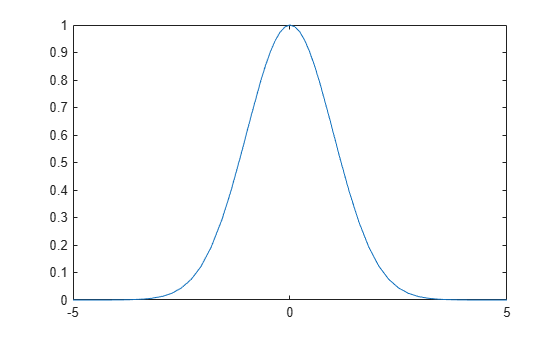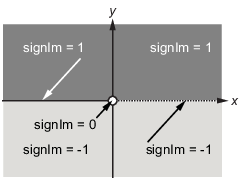## Integration

If `f` is a symbolic expression, then

`int(f)`

attempts to find another symbolic expression, `F`, so that `diff(F)` `=` `f`. That is, `int(f)` returns the indefinite integral or antiderivative of `f` (provided one exists in closed form). Similar to differentiation,

`int(f,v)`

uses the symbolic object `v` as the variable of integration, rather than the variable determined by `symvar`. See how `int` works by looking at this table.

Mathematical Operation

MATLAB® Command

`int(x^n)` or `int(x^n,x)`

$\underset{0}{\overset{\pi /2}{\int }}\mathrm{sin}\left(2x\right)dx=1$

`int(sin(2*x), 0, pi/2)` or ```int(sin(2*x), x, 0, pi/2)```

g  =  cos(at + b)

$\int g\left(t\right)dt=\mathrm{sin}\left(at+b\right)/a$

`g = cos(a*t + b) int(g)` or ```int(g, t)```

$\int {J}_{1}\left(z\right)dz=-{J}_{0}\left(z\right)$

`int(besselj(1, z))` or ```int(besselj(1, z), z)```

In contrast to differentiation, symbolic integration is a more complicated task. A number of difficulties can arise in computing the integral:

• The antiderivative, `F`, may not exist in closed form.

• The antiderivative may define an unfamiliar function.

• The antiderivative may exist, but the software can't find it.

• The software could find the antiderivative on a larger computer, but runs out of time or memory on the available machine.

Nevertheless, in many cases, MATLAB can perform symbolic integration successfully. For example, create the symbolic variables

`syms a b theta x y n u z`

The following table illustrates integration of expressions containing those variables.

f

int(f)

```syms x n f = x^n;```
`int(f)`
```ans = piecewise(n == -1, log(x), n ~= -1,... x^(n + 1)/(n + 1))```
```syms y f = y^(-1);```
`int(f)`
```ans = log(y)```
```syms x n f = n^x;```
`int(f)`
```ans = n^x/log(n)```
```syms a b theta f = sin(a*theta+b);```
`int(f)`
```ans = -cos(b + a*theta)/a```
```syms u f = 1/(1+u^2);```
`int(f)`
```ans = atan(u)```
```syms x f = exp(-x^2);```
`int(f)`
```ans = (pi^(1/2)*erf(x))/2```

In the last example, `exp(-x^2)`, there is no formula for the integral involving standard calculus expressions, such as trigonometric and exponential functions. In this case, MATLAB returns an answer in terms of the error function `erf`.

If MATLAB is unable to find an answer to the integral of a function `f`, it just returns `int(f)`.

Definite integration is also possible.

Definite Integral

Command

`${\int }_{a}^{b}f\left(x\right)dx$`

`int(f, a, b)`

`${\int }_{a}^{b}f\left(v\right)dv$`

`int(f, v, a, b)`

f

a, b

int(f, a, b)

```syms x f = x^7;```
```a = 0; b = 1;```
`int(f, a, b)`
```ans = 1/8```
```syms x f = 1/x;```
```a = 1; b = 2;```
`int(f, a, b)`
```ans = log(2)```
```syms x f = log(x)*sqrt(x);```
```a = 0; b = 1;```
`int(f, a, b)`
```ans = -4/9```
```syms x f = exp(-x^2);```
```a = 0; b = inf;```
`int(f, a, b)`
```ans = pi^(1/2)/2```
```syms z f = besselj(1,z)^2;```
```a = 0; b = 1;```
`int(f, a, b)`
```ans = hypergeom([3/2, 3/2],... [2, 5/2, 3], -1)/12```

For the Bessel function (`besselj`) example, it is possible to compute a numerical approximation to the value of the integral, using the `double` function. The commands

```syms z a = int(besselj(1,z)^2,0,1)```

return

```a = hypergeom([3/2, 3/2], [2, 5/2, 3], -1)/12```

and the command

`a = double(a)`

returns

```a = 0.0717```

### Integration with Real Parameters

One of the subtleties involved in symbolic integration is the “value” of various parameters. For example, if a is any positive real number, the expression

`${e}^{-a{x}^{2}}$`

is the positive, bell shaped curve that tends to 0 as x tends to ±∞. You can create an example of this curve, for a = 1/2.

```syms x a = sym(1/2); f = exp(-a*x^2); fplot(f)```However, if you try to calculate the integral

`$\underset{-\infty }{\overset{\infty }{\int }}{e}^{-a{x}^{2}}dx$`

without assigning a value to a, MATLAB assumes that a represents a complex number, and therefore returns a piecewise answer that depends on the argument of a. If you are only interested in the case when a is a positive real number, use `assume` to set an assumption on `a`:

```syms a assume(a > 0)```

Now you can calculate the preceding integral using the commands

```syms x f = exp(-a*x^2); int(f, x, -inf, inf)```

This returns

```ans = pi^(1/2)/a^(1/2)```

### Integration with Complex Parameters

To calculate the integral

`$\underset{-\infty }{\overset{\infty }{\int }}\frac{1}{{a}^{2}+{x}^{2}}\text{ }dx$`

for complex values of `a`, enter

```syms a x f = 1/(a^2 + x^2); F = int(f, x, -inf, inf)```

Use `syms` to clear all the assumptions on variables. For more information about symbolic variables and assumptions on them, see Delete Symbolic Objects and Their Assumptions.

The preceding commands produce the complex output

```F = (pi*signIm(1i/a))/a```

The function `signIm` is defined as:To evaluate `F` at `a = 1 + i`, enter

`g = subs(F, 1 + i)`
```g = pi*(1/2 - 1i/2)```
`double(g)`
```ans = 1.5708 - 1.5708i```

### High-Precision Numerical Integration Using Variable-Precision Arithmetic

High-precision numerical integration is implemented in the `vpaintegral` function of the Symbolic Math Toolbox™. `vpaintegral` uses variable-precision arithmetic in contrast to the MATLAB `integral` function, which uses double-precision arithmetic.

Integrate `besseli(5,25*u).*exp(-u*25)` by using both `integral` and `vpaintegral`. The `integral` function returns `NaN` and issues a warning while `vpaintegral` returns the correct result.

```syms u f = besseli(5,25*x).*exp(-x*25); fun = @(u)besseli(5,25*u).*exp(-u*25); usingIntegral = integral(fun, 0, 30) usingVpaintegral = vpaintegral(f, 0, 30) ```
```Warning: Infinite or Not-a-Number value encountered. usingIntegral = NaN usingVpaintegral = 0.688424 ```

For more information, see `vpaintegral`.